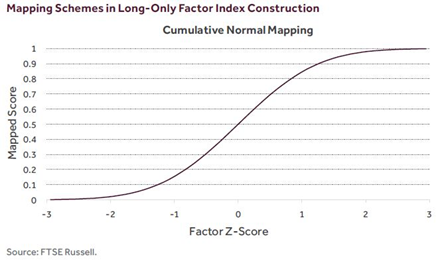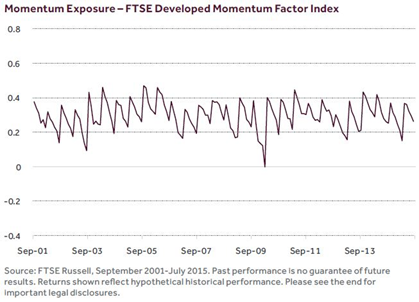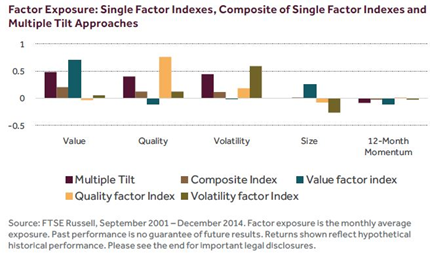An equity factor index is intended to offer controlled exposure to a factor or factors. But how does it achieve this goal in practice? There are a number of conceptual and design steps involved in the creation of an equity factor index and in this paper we explore these decisions.

Long/short and long-only factor indexes

A factor index is a collection of securities constructed to mimic the behaviour of a particular factor. For example, a size factor index is designed to capture the risk premium attributable to the size factor, something it achieves by holding smaller-capitalisation stocks. A value factor index reflects the performance of the value factor risk premium; in this case, by holding stocks that score highest according to a value measure, such as the book -to-price ratio or earnings yield.

In their purest, theoretical form, factor indexes are long/short: in other words, negative index weightings (short positions) as well as positive weightings (long positions) are permitted. A simple long/short size factor index could therefore be long small-capitalisation stocks and short large-capitalisation stocks. A long/short value factor index might be long value (low P/B or P/E) stocks and short growth (high P/B or P/E) stocks.

In practice, many investors are unable to short stocks and standard factor indexes are designed to reflect this long-only constraint.

In a 2014 paper, researchers from Robeco Asset Management compared long/ short and long-only factor investing approaches and argued that, although a long/short approach is theoretically superior, practical issues such as benchmark restrictions and implementation costs mean that a long-only approach to index construction is the preferred alternative for most investors1.

Converting factor scores to a factor index

Within a universe of stocks, factor scores are standardised by subtracting the mean score and dividing the result by the standard deviation of the universe of factor scores.

There are different methods for converting these standardised factor scores (or “Z-Scores”), which have both negative and positive values, into a set of positive scores that can be used to calculate index weights.

In the FTSE Global Factor Index Series, FTSE Russell’s approach is to use the cumulative normal distribution function for this purpose. This approach limits the effect of the smallest and largest factor scores within the sample and avoids a potentially problematic concentration of the index in stocks with high factor scores.Maintaining factor exposure

Over time, the factor exposure of a factor index is subject to decay. Effectively, once the factor index is constituted, mean reversion causes the absolute factor scores of individual stocks to converge steadily, leading to a gradual weakening of the index’s factor score.

This is evident in the chart below, which shows the exposure of the FTSE Developed Momentum Factor index between 2001 and 2014 to momentum. The saw-tooth pattern of the factor exposure chart reflects the decay in the exposure between index rebalancings, followed by a jump in the exposure each time the index is rebalanced (in this case, semi-annually).

The speed of the decay of the index’s factor signal also depends on the factor itself: some factors (for example, size, liquidity and quality) exhibit less pronounced factor decay3. In turn, this impacts the rebalancing frequency chosen by the index provider. Within FTSE’s Global Factor index series, rebalancing frequencies are either annual or semi-annual.Combining factors

Investors frequently wish to combine factors in their portfolios. According to the 2015 FTSE Russell smart beta survey, over 70% of respondents with a current allocation to smart beta, a broad category that includes factor indexes, are using two or more smart beta strategies.

However, there are different ways of combining factors using indexes. A simple approach is to average stock weights across the desired single factor indexes. A variant of this approach is to use a composite of the target factors to create a single composite factor index.

While this approach works well for factors that are positively correlated, if factors are negatively correlated an averaging/composite approach may dilute exposure to the desired factors.

An alternative approach to achieving exposure to multiple factors within an index is to “tilt” the starting index repeatedly, each time towards one of the desired factors. In other words, index weights are first tilted towards the first factor of interest, then towards a second factor of interest, and so on. The multiple tilt procedure allows the index designer to retain exposure to all the desired factors.

In the following chart we illustrate the difference between the averaging/ composite approach and the multiple tilt approach by examining the levels of factor exposure when three factors (value, quality and volatility) are combined.

By comparison with the target factor exposure of each of the single factor indexes (e.g., the value factor exposure of the value factor index), the exposure of the composite index (shown by the grey bar) is much reduced. By contrast, the multiple tilt approach (the red bar) preserves much of the factor exposure of the three factors being combined.

The dilution of the factor exposure of a composite factor index is a consequence of the tendency of value and quality factors to be negatively correlated.

The size and twelve month momentum factor exposures of the three single factor indexes, the composite index and the multiple tilt approach are also shown for purposes of comparison.Factor indexes

Factor indexes are transparent, rules-based baskets of securities that target controlled and consistent exposure to factors over time. They are designed to be used both as performance benchmarks in asset management and as the basis for index-replicating financial products. While the financial theory underlying factors often assumes that investors can as easily obtain short as long portfolio positions, in practice most factor indexes are designed for use in a long-only context.

Converting factor scores into index weightings requires the choice of a mapping procedure. In the FTSE Global Factor Index Series, FTSE Russell uses a mapping approach that dampens the effect of the smallest and largest factor scores within the sample. Factor indexes require regular rebalancing to maintain their factor exposure, with the rebalancing frequency chosen to reflect the characteristics of the factor(s) concerned.

There are different approaches to combining factors. A simple approach of averaging weights across factor indexes, or of creating a composite factor index from individual factors, may work well if factors are positively correlated. However, if factors work in opposition to each other, combining factors in this way may lead to a dilution of the desired factor exposure. An alternative approach, multiple tilting, allows the index designer to retain exposure to multiple factors by the repeated application of a tilting process towards the factors of interest.